Courses

# Equilibrium of a Rigid Body Class 11 Notes | EduRev

## Class 11 : Equilibrium of a Rigid Body Class 11 Notes | EduRev

The document Equilibrium of a Rigid Body Class 11 Notes | EduRev is a part of the Class 11 Course Physics Class 11.
All you need of Class 11 at this link: Class 11

6. Body is in equilibrium : -

We can say rigid body is in equillibrium when it is in

(a) Translational equilibrium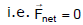Fnet x = 0 and Fnet y = 0 and

(b) Rotational equilibrium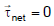Note :

(i) If net force on the body is zero then net torque of the forces may or may not be zero.

example.

A pair of forces each of same magnitude and acting in opposite direction on the rod.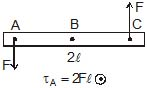(2) If net force on the body is zero then torque of the forces about each and every point is same

t about B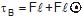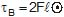t about C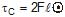Ex.18 Determine the point of application of third force for which body is in equillibrium when forces of 20 N & 30 N are acting on the rod as shown in figure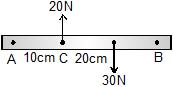Sol. Let the magnitude of third force is F, is applied in upward direction then the body is in the equilibrium when

(i)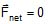(Translational Equillibrium)

⇒ 20 + F = 30 ⇒ F = 10 N

So the body is in translational equilibrium when 10 N force act on it in upward direction.

(ii)

Let us assume that this 10 N force act. Then keep the body in rotational equilibrium So Tor que about C = 0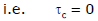30 x 20 = 10 x
x = 60 cm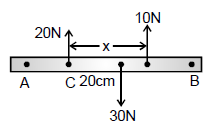so 10 N force is applied at 70 cm from point A to keep the body in equilibrium.

Ex.19 Determine the point of application of force, when forces are acting on the rod as shown in figure.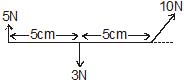Sol. Since the body is in equillibrium so we conclude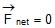and torque about any point is zero i.e.,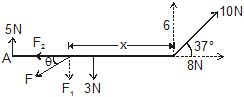Let us assume that we apply F force downward at A angle q from the horizontal, at x distance from B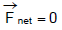⇒ Fnet x = 0 which gives
F2 = 8 N
From Fnet y = 0 ⇒ 5 + 6 = F+ 3
⇒ F1 = 8 N
If body is in equilibrium then torque about point B is zero,
⇒    3 x 5 + F1 x - 5 x 10 = 0
⇒    15 + 8x - 50 = 0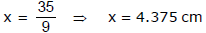Ex.20 A uniform rod length l, mass m is hung from two strings of equal length from a ceiling as shown in figure. Determine the tensions in the strings ?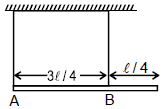Sol. Let us assume that tension in left and right string is TA and TB respectively. Then

Rod is in equilibrium then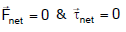From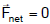mg = TA + TB ...(1)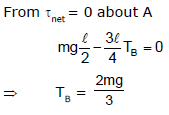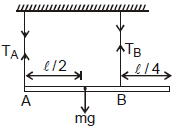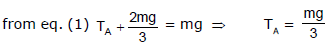Ex.21 A stationary uniform rod of mass `m', length `l' leans against a smooth vertical wall making an angle q with rough horizontal floor. Find the normal force & frictional force that is exerted by the floor on the rod?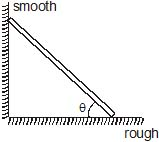Sol. As the rod is stationary so the linear acceleration and angular acceleration of rod is zero.

Offer running on EduRev: Apply code STAYHOME200 to get INR 200 off on our premium plan EduRev Infinity!

## Physics Class 11

75 videos|312 docs|152 tests

,

,

,

,

,

,

,

,

,

,

,

,

,

,

,

,

,

,

,

,

,

;